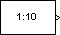# Signal From Workspace

Import signal from MATLAB workspace

•Libraries:
DSP System Toolbox / Sources

## Description

The Signal From Workspace block imports a signal from the MATLAB® workspace into the Simulink® model. The Signal parameter specifies the name of a MATLAB workspace variable containing the signal to import, or any valid MATLAB expression defining a matrix or 3-D array.

Unlike the Simulink From Workspace (Simulink) block, the Signal From Workspace block holds the output value constant between successive output frames (that is, no linear interpolation takes place). Also, the initial signal values are always produced immediately at t=0.

## Ports

### Output

expand all

Signal imported from workspace, as a scalar, vector, matrix, or 3-D array.

When the Signal parameter specifies an M-by-N matrix (M≠1), each of the N columns is treated as a distinct channel. You specify the frame size in the Samples per frame parameter, Mo. The output is an Mo-by-N matrix containing Mo consecutive samples from each signal channel. You specify the output sample period in the Sample time parameter, Ts, and the output frame period is MoTs. For convenience, an imported row vector (M=1) is treated as a single channel, so the output dimension is Mo-by-1.

When the Signal parameter specifies an M-by-N-by-P array, each of the P pages (an M-by-N matrix) is output in sequence with period Ts. The Samples per frame parameter must be set to `1`.

Data Types: `single` | `double` | `int8` | `int16` | `int32` | `int64` | `uint8` | `uint16` | `uint32` | `uint64` | `fixed point`

## Parameters

expand all

The name of the MATLAB workspace variable from which to import the signal, or a valid MATLAB expression specifying the signal.

The sample period, Ts, of the output, specified as a scalar or vector. The output frame period is MoTs.

The number of samples, Mo, to buffer into each output frame, specified as a positive integer scalar. This value must be `1` when you specify a 3-D array in the Signal parameter.

Specifies the output after all of the specified signal samples have been generated.

• When you specify `Setting To Zero`, the block generates zero-valued outputs for the duration of the simulation after generating the last frame of the signal.

• When you specify `Holding Final Value`, the block repeats the final sample for the duration of the simulation after generating the last frame of the signal.

• When you specify `Cyclic Repetition`, the block repeats the signal from the beginning after it reaches the last sample in the signal. If the frame size you specify in the Samples per frame parameter does not evenly divide the input length, a buffer block is inserted into the Signal From Workspace subsystem, and the model becomes multirate. If you do not want your model to become multirate, make sure that the frame size evenly divides the input signal length.

The block does not extrapolate the imported signal beyond the last sample.

Select the Warn when frame size does not evenly divide input length parameter to be alerted when the input length is not an integer multiple of the frame size. When the input length is not an integer multiple of the frame size, the model becomes multirate. Use the Model Explorer to turn these warnings on or off model-wide:

1. In the Modeling tab, click .

2. In the Search bar of the Model Explorer, search ```by Property Name``` for the `ignoreOrWarnInputAndFrameLengths` property. Each block with the Warn when frame size does not evenly divide input length check box appears in the list in the Contents pane.

3. Select each of the blocks for which you want to toggle the warning parameter, and select or clear the check box in the `ignoreOrWarnInputAndFrameLengths` column.

#### Dependencies

To enable this parameter set Form output after final data value by to ```Cyclic Repetition```.

## Block Characteristics

 Data Types `double` | `fixed point` | `integer` | `single` Direct Feedthrough `no` Multidimensional Signals `no` Variable-Size Signals `no` Zero-Crossing Detection `no`

## Version History

Introduced before R2006a